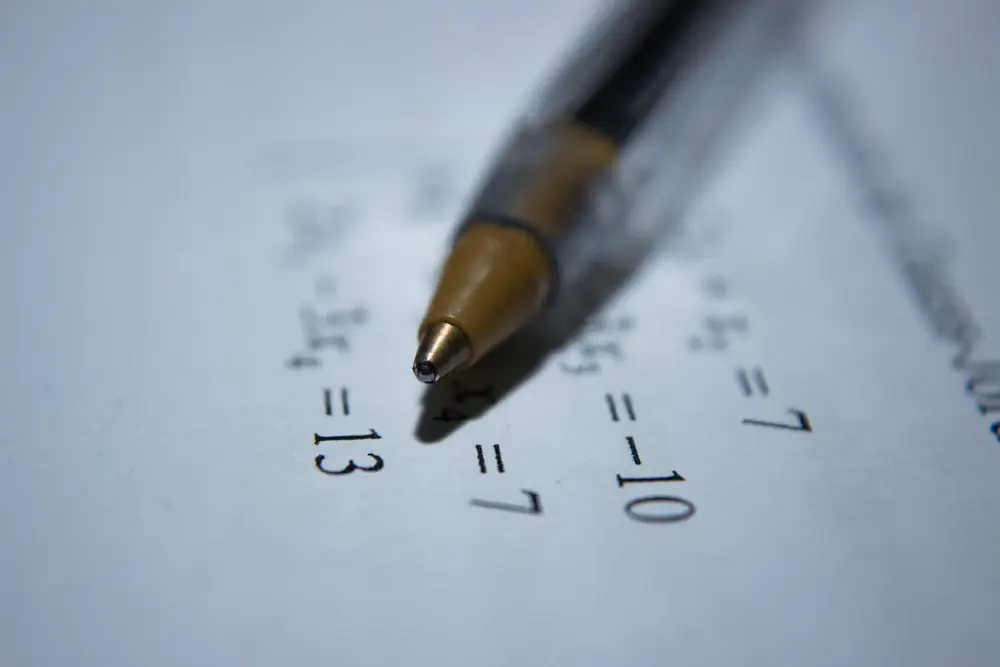# Sequences

#### Introduction

• In the sequence 2, 4, 6, 8, 10... there is an obvious pattern. Such sequences can be expressed in terms of the nth term of the sequence. In this case, the nth term = 2n. To find the 1st term, put n = 1 into the formula, to find the 4th term, replace the n's by 4's: 4th term = 2 × 4
• = 8.
• Example:
• What is the nth term of the sequence 2, 5, 10, 17, 26... ?
• n = 1 2 3 4 5
• n² = 1 4 9 16 25
• n² + 1 = 2 5 10 17 26
• This is the required sequence, so the nth term is n² + 1. For some sequences, there is no easy way of working out the nth term of a sequence, other than to try different possibilities.
• Tips: if the sequence is going up in threes (e.g. 3, 6, 9, 12...), there will probably be a three in the formula, etc (see arithmetic progressions below).
• In many cases, square numbers will come up, so try squaring n, as above. Also, the triangular numbers formula often comes up. This is n(n + 1)/2 .
• Example:
• Find the nth term of the sequence: 2, 6, 12, 20, 30...
• n = 1 2 3 4 5
• n(n + 1)/2 = 1 3 6 10 15
• Clearly the required sequence is double the one we have found the nth term for, therefore the nth term of the required sequence is 2n(n+1)/2 = n(n + 1).

#### Notation

• The nth term of a sequence is usually written as Un . S
• o in the last example, Un = n(n + 1) .
• The 5th term is therefore U5 = 5(5 + 1) = 30.

#### Recurrence relation

• This is where the next term of a sequence is defined using the previous term(s).
• For example, the recurrence relation for 2, 4, 8, 16, 32, ... would be: U1 = 2, Un = 2(Un-1).
• This tells us that the first term, U1, is 2 and the next term of the sequence can be found by doubling the previous term.

#### Convergent Sequences

• Sequences whose nth term approaches a finite number as n becomes larger are known as convergent sequences and the number to which the sequence converges is known as the limit of the sequence.
• For example: 10, 5, 2.5, 1.25, 0.625, ... converges towards the limit zero.
Algebra

Algebra

Algebra

Algebra

Algebra

Algebra